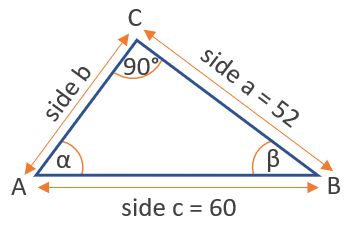# Arcsine Calculator

Use this arcsine calculator to easily calculate the arcsine of a number. Online arcsine calculation tool with output in degrees or radians. Supports input of decimal numbers (0.5, 6, -1, etc.) and fractions (1/4, 2/3, 4/3, 1/3 etc.).

### Calculation results

30 degrees arcsin(0.5) = arcsin(0.5) =
Share calculator:

Embed this tool:
get code

## Arcsine function

The arcsine is one of the inverse trigonometric functions (antitrigonometric functions) and is the inverse of the sine function. It is sometimes written as sin-1(x), but this notation should be avoided as it can be confused with an exponent notation (power of, raised to the power of). The arcsine is used to obtain an angle from the sine trigonometric ratio, which is the ratio between the side opposite to the angle and the longest side of the triangle.

The function spans from -1 to 1, and so do the results from our arcsin calculator. The range of the angle values is usually between -90° and 90°. There are a number of arcsin rules, like that sin(arcsin(x)) = x, or that arcsinα + arcsinβ = arcsin(α√(1-β2) + β√(1-α2)), as well as cosine of the arcsine: cos(arcsin(x)) = sin(arccos(x)) = √(1-x2), which can help you in trigonometry calculus.

## How to calculate the arcsine of a number?

The easiest way to calculate it is by using our arcsin calculator above, which will output results in both degrees and radians. Other ways include other given information, such as the values of other trigonometric functions for the same angle or other angles in the same triangle.

Here is a table of common arcsin values:

Common values of the arcsin function
-1 -90° -π/2
-√3 / 2 -60° -π/3
-√2 / 2 -45° -π/4
-1/2 -30° -π/6
0 0
1/2 30° π/6
√2 / 2 45° π/4
√3 / 2 60° π/3
1 90° π/2

π is, of course, the mathematical constant about equal to 3.14159.

## Using arcsine to find an angle

Given the right angled triangle in the figure below with known length of side a = 52 and of the hypotenuse c = 60 the inverse cosine function arcsin can be used to find the angle α at point A.First, calculate the sine of α by dividng the opposite side by the hypotenuse. This results in sin(α) = a / c = 52 / 60 = 0.8666. Use the inverse function with this outcome to calculate the angle α = arcsin(0.8666) = 60° (1.05 radians).

#### Cite this calculator & page

If you'd like to cite this online calculator resource and information as provided on the page, you can use the following citation:
Georgiev G.Z., "Arcsin Calculator", [online] Available at: https://www.gigacalculator.com/calculators/arcsin-calculator.php URL [Accessed Date: 27 Mar, 2023].